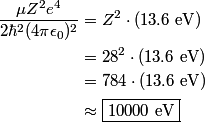## Solution to 1986 Problem 26

 K series x-rays are produced when an electron in the K ($n$ = 1) shell of one of the target atoms is knocked out of the atom by one of the fast electrons. The electrons in the K shell of nickel are bound by an energy of\begin{align*}\frac{\mu Z^2 e^4}{2 \hbar^2 (4 \pi \epsilon_0)^2} &= Z^2 \cdot \left(13.6 \mbox{ eV}\right) \\&= 28^2 ...This is the energy that the fast electron must have to knock out a K shell electron. Therefore, answer (D) is correct.